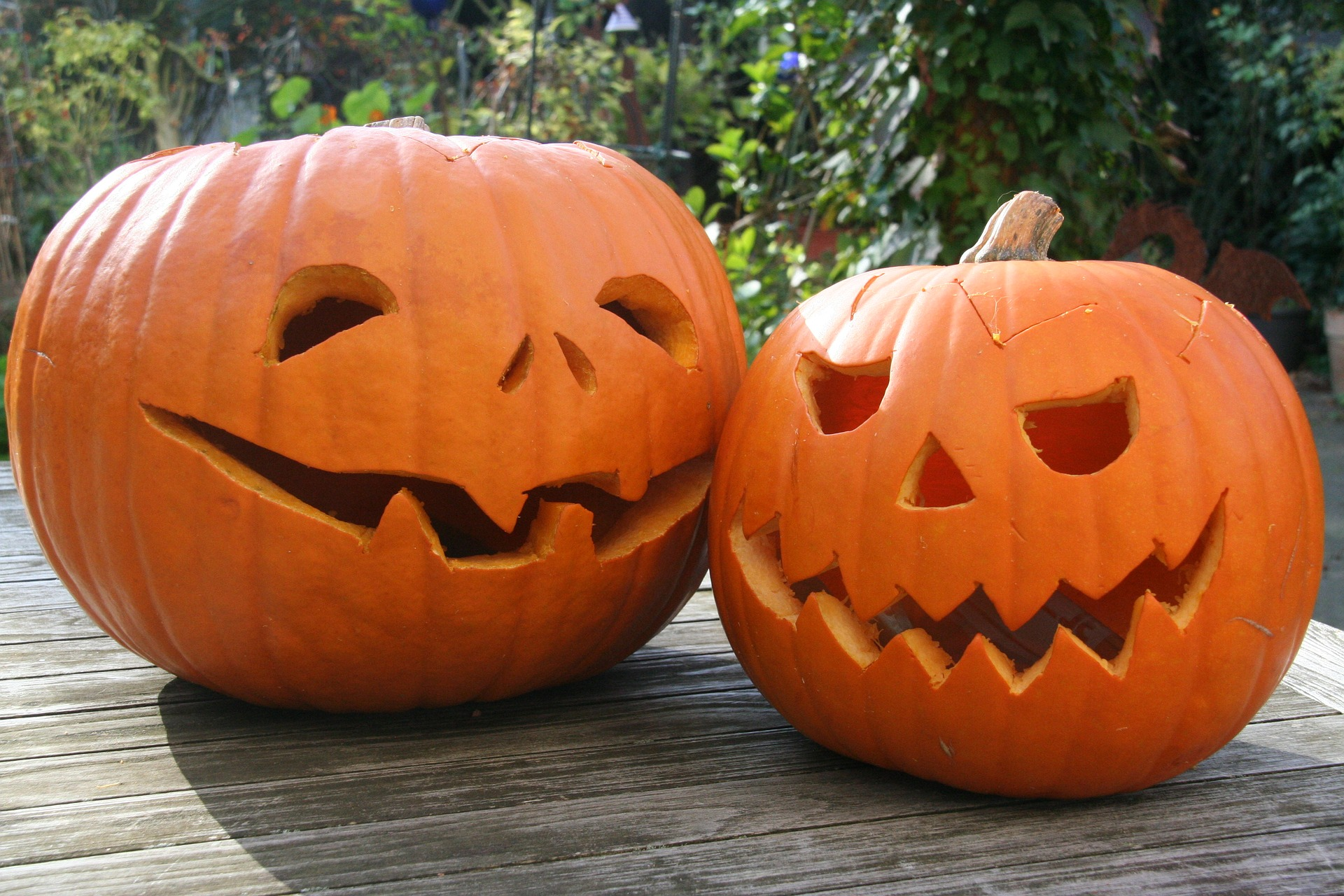## Putting It Together: The Language of Algebra

Let’s revisit the scenario from the beginning of the module where Lydia Lee was about to calculate how much each person in her family could spend at the the Badger Hill Farm. When Aubrey and Maya’s parents told the girls about carving pumpkins and eating caramel apples, they got super excited and wanted to know what else they can do while they are visiting the farm!So far, these are the expenses for the trip:

Lodging: 3 nights at an apartment rental at $180/night $180\cdot{3}=\540$ Food: 3 days of groceries and restaurant meals at$75/day for the whole family

$75\cdot{3}=\225$

Science Center: 2 adult tickets and 2 child tickets, the adult tickets are $24 each and the child tickets are$19 each

$24\cdot{2}=\48$ (adult tickets)

$19\cdot{2}=\38$ (child tickets)

Badger Hill Farm: 4 tickets for entry to the farm during the autumn fair

$8\cdot{4}=\32$ (entry tickets)

If we add up all the known expenses, the expression will be:

lodging + food + museum tickets + farm tickets

$540+225+48+38+32$

$=\883$

The unknown in this case, or variable, is how much each person has to spend on extras at the farm. We’ll call this amount $x$. There are four people in the family, so the total they will be able to spend is $4x$. Now let’s help Lydia write an equation to solve for the value of $x$. She knows it will have to look like this if they are going to stay on budget:

cost of known amounts  +  extra spending at farm  =  total amount budgeted

$883+4x=955$

$4x=72$

$x=18$

Each member of the Lee family can spend $18 on extra fun. Maya wants to carve a pumpkin and eat a caramel apple and wonders how much money she will have left over to save for her weekly sticker shopping spree. The caramel apples cost$5 each and the pumpkin carving is $12, so the equation to determine the leftover money must be: $12+5+z=18$ $z=1$ Maya will have$1 left over to buy stickers.

Aubrey wants to go in the corn maze and then get as many bags of popcorn as possible. The corn maze is $10 and each bag of popcorn is$4, so the equation to determine how many bags of popcorn must be:

$10+4y=18$

$y=2$

Aubrey can buy 2 bags of popcorn and go in the corn maze.

As we demonstrated in these examples, algebra is used in all sorts of common everyday calculations that you make. After making progress through more complex math topics, you will also be able to solve complicated real world problems that involve algebra.

## Contribute!

Did you have an idea for improving this content? We’d love your input.